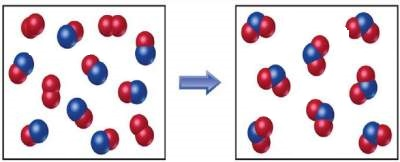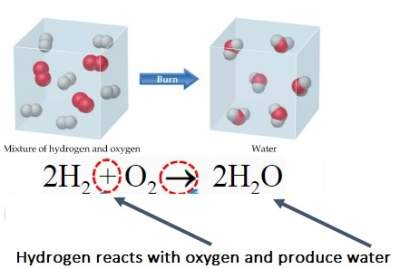# Introduction to Stoichiometry

## Introduction

Stoichiometry is built on the understanding of atomic masses and the law of conservation of mass. See illustration below:## Law of Conservation of Mass

"Atoms are neither created nor destroyed during any chemical reaction".
So, what happens during a chemical reaction?! ⇒ Rearrangements of the atoms involved in the reaction.

### Example

Burning of hydrogen in air is depicted in the figure below:So, the reactions 2H2 + O2 → Products 2H2O

By applying the law of conservation of mass, a chemical Equation must have an equal number of atoms of each element on each side of the arrow i.e. "Balanced Equation"